GiNaC 1.8.7
GiNaC::multi_iterator_permutation< T > Class Template Reference

The class multi_iterator_permutation defines a multi_iterator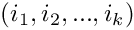, for which. More...

#include <utils_multi_iterator.h>

Inheritance diagram for GiNaC::multi_iterator_permutation< T >: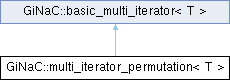## Public Member Functions

multi_iterator_permutation (void)
Default constructor. More...

multi_iterator_permutation (T B, T N, size_t k)
Construct a multi_iterator with upper limit N and size k . More...

multi_iterator_permutation (T B, T N, const std::vector< T > &vv)
Construct from a vector. More...

basic_multi_iterator< T > & init (void)
Initialize the multi-index to. More...

basic_multi_iterator< T > & operator++ (int)
The postfix increment operator allows to write for a multi-index n++, which will update n to the next configuration. More...

int get_sign (void) const
Returns the sign of the permutation, defined by. More...Public Member Functions inherited from GiNaC::basic_multi_iterator< T >
basic_multi_iterator (void)
Default constructor. More...

basic_multi_iterator (T B, T N, size_t k)
Construct a multi_iterator with upper limit N, lower limit B and size k . More...

basic_multi_iterator (T B, T N, const std::vector< T > &vv)
Construct from a vector. More...

virtual ~basic_multi_iterator ()
Destructor. More...

size_t size (void) const
Returns the size of a multi_iterator. More...

bool overflow (void) const
Return the overflow flag. More...

const std::vector< T > & get_vector (void) const
Returns a reference to the vector v. More...

operator[] (size_t i) const
Subscription via []. More...

T & operator[] (size_t i)
Subscription via []. More...

operator() (size_t i) const
Subscription via () More...

T & operator() (size_t i)
Subscription via () More...

virtual basic_multi_iterator< T > & init (void)
Initialize the multi-index to. More...

virtual basic_multi_iterator< T > & operator++ (int)
No effect for basic_multi_iterator. More...

## Friends

template<class TT >
std::ostream & operator<< (std::ostream &os, const multi_iterator_permutation< TT > &v)Protected Attributes inherited from GiNaC::basic_multi_iterator< T >
N

B

std::vector< T > v

bool flag_overflow

## Detailed Description

template<class T>
class GiNaC::multi_iterator_permutation< T >

The class multi_iterator_permutation defines a multi_iterator, for which.and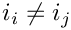In particular, if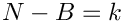, multi_iterator_permutation loops over all permutations of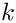elements.

Definition at line 321 of file utils_multi_iterator.h.

## ◆ multi_iterator_permutation() [1/3]

template<class T >
 GiNaC::multi_iterator_permutation< T >::multi_iterator_permutation ( void )
inline

Default constructor.

Definition at line 1119 of file utils_multi_iterator.h.

## ◆ multi_iterator_permutation() [2/3]

template<class T >
 GiNaC::multi_iterator_permutation< T >::multi_iterator_permutation ( T B, T N, size_t k )
inlineexplicit

Construct a multi_iterator with upper limit N and size k .

Definition at line 1127 of file utils_multi_iterator.h.

## ◆ multi_iterator_permutation() [3/3]

template<class T >
 GiNaC::multi_iterator_permutation< T >::multi_iterator_permutation ( T B, T N, const std::vector< T > & vv )
inlineexplicit

Construct from a vector.

Definition at line 1135 of file utils_multi_iterator.h.

## ◆ init()

template<class T >
 basic_multi_iterator< T > & GiNaC::multi_iterator_permutation< T >::init ( void )
inlinevirtual

Initialize the multi-index to.Reimplemented from GiNaC::basic_multi_iterator< T >.

Definition at line 1148 of file utils_multi_iterator.h.

## ◆ operator++()

template<class T >
 basic_multi_iterator< T > & GiNaC::multi_iterator_permutation< T >::operator++ ( int )
inlinevirtual

The postfix increment operator allows to write for a multi-index n++, which will update n to the next configuration.

If n is in the last configuration and the increment operator ++ is applied to n, the overflow flag will be raised.

Reimplemented from GiNaC::basic_multi_iterator< T >.

Definition at line 1171 of file utils_multi_iterator.h.

References k.

## ◆ get_sign()

template<class T >
 int GiNaC::multi_iterator_permutation< T >::get_sign ( void ) const
inline

Returns the sign of the permutation, defined by.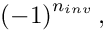where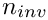is the number of inversions, e.g. the number of pairs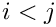for which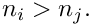Definition at line 1242 of file utils_multi_iterator.h.

References k.

## ◆ operator<<

template<class T >
template<class TT >
 std::ostream & operator<< ( std::ostream & os, const multi_iterator_permutation< TT > & v )
friend

The documentation for this class was generated from the following file:

This page is part of the GiNaC developer's reference. It was generated automatically by doxygen. For an introduction, see the tutorial.